• Portfolio
• Stream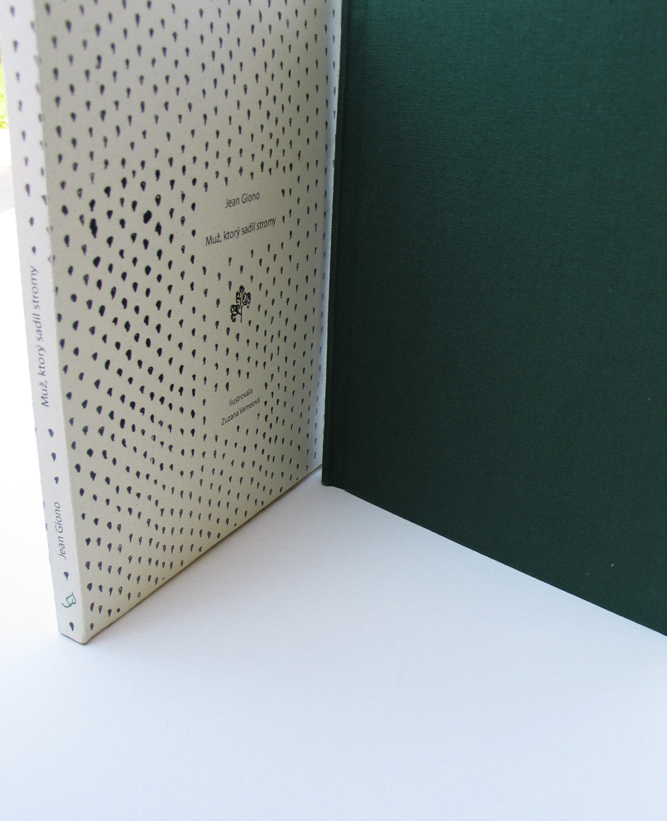detail of the cover - The idea of illustration in this book was follow the simple but strong tale: to grow,evolve and intesify with a story of book. As u can see on a first spreads, there are just seeds that at the end turned in to the forest, so the illustration does.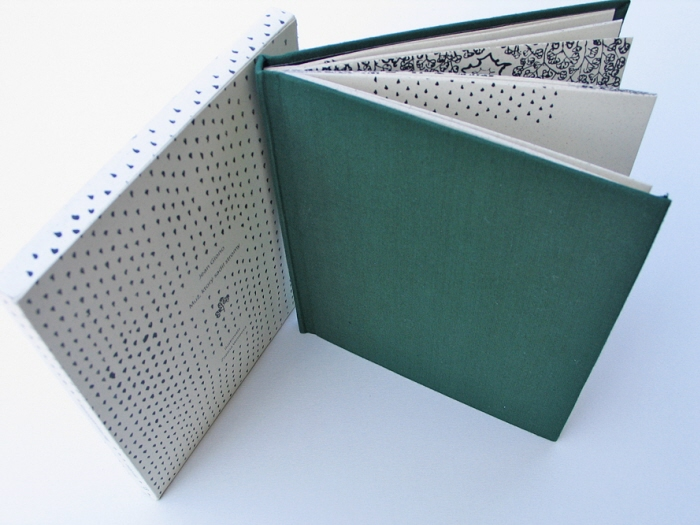the book cover - The idea of illustration in this book was follow the simple but strong tale: to grow,evolve and intesify with a story of book. As u can see on a first spreads, there are just seeds that at the end turned in to the forest, so the illustration does.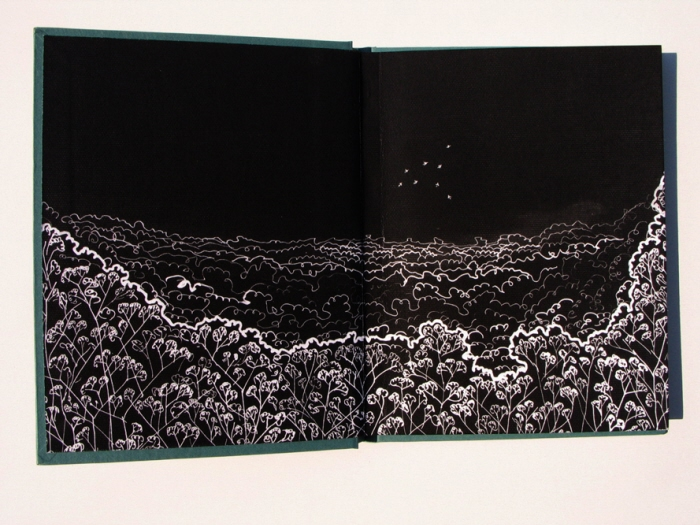the endpaper of the book - The idea of illustration in this book was follow the simple but strong tale: to grow,evolve and intesify with a story of book. As u can see on a first spreads, there are just seeds that at the end turned in to the forest, so the illustration does.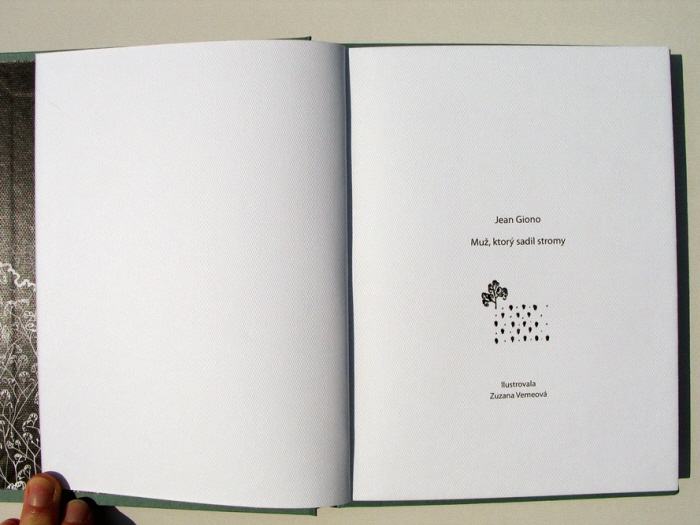the titul - The idea of illustration in this book was follow the simple but strong tale: to grow,evolve and intesify with a story of book. As u can see on a first spreads, there are just seeds that at the end turned in to the forest, so the illustration does.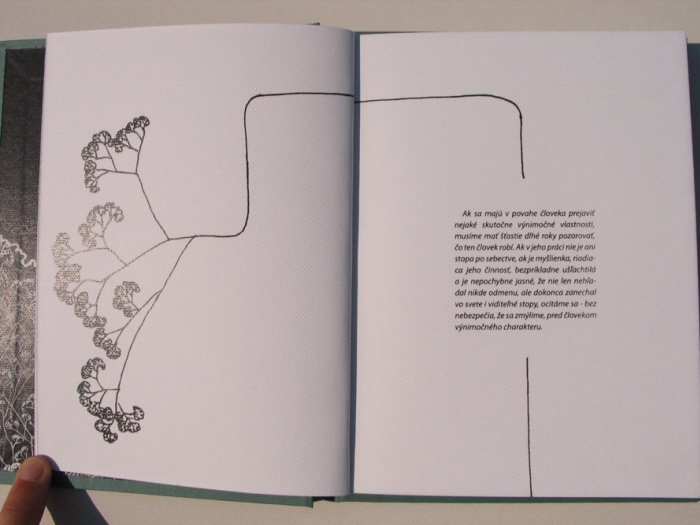spread - The idea of illustration in this book was follow the simple but strong tale: to grow,evolve and intesify with a story of book. As u can see on a first spreads, there are just seeds that at the end turned in to the forest, so the illustration does.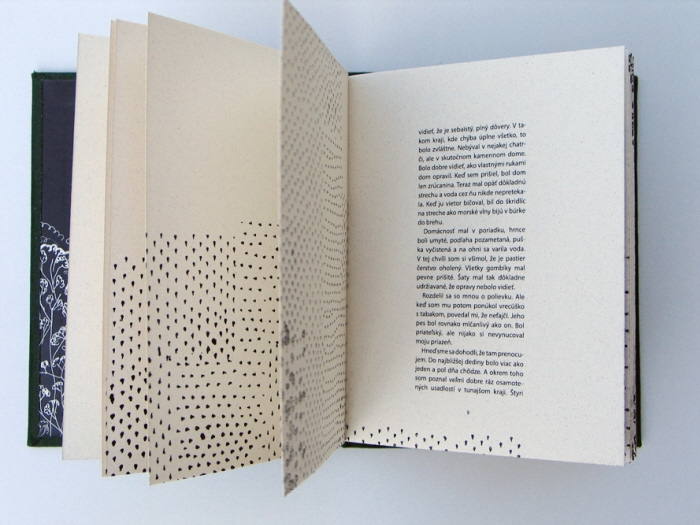spread - The idea of illustration in this book was follow the simple but strong tale: to grow,evolve and intesify with a story of book. As u can see on a first spreads, there are just seeds that at the end turned in to the forest, so the illustration does.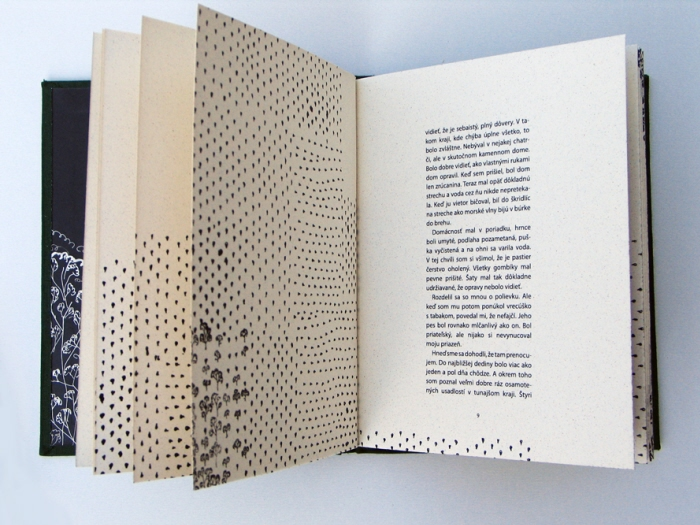spread - The idea of illustration in this book was follow the simple but strong tale: to grow,evolve and intesify with a story of book. As u can see on a first spreads, there are just seeds that at the end turned in to the forest, so the illustration does.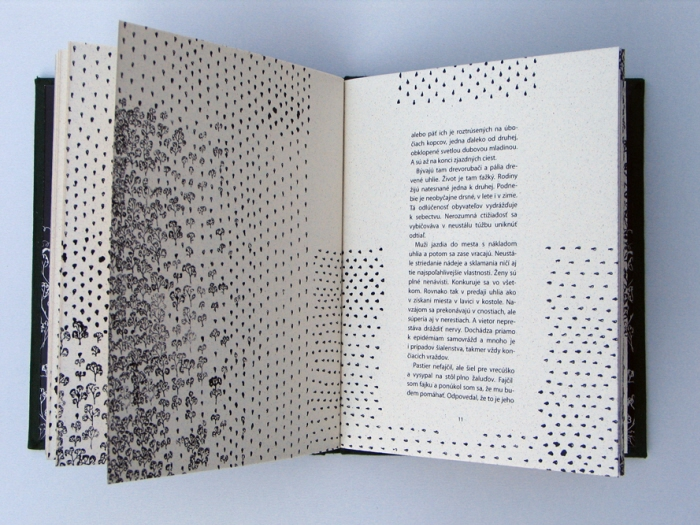spread - The idea of illustration in this book was follow the simple but strong tale: to grow,evolve and intesify with a story of book. As u can see on a first spreads, there are just seeds that at the end turned in to the forest, so the illustration does.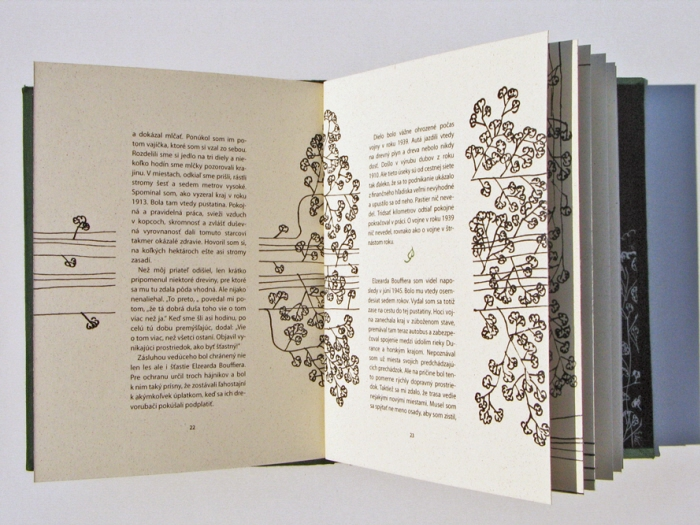spread - The idea of illustration in this book was follow the simple but strong tale: to grow,evolve and intesify with a story of book. As u can see on a first spreads, there are just seeds that at the end turned in to the forest, so the illustration does.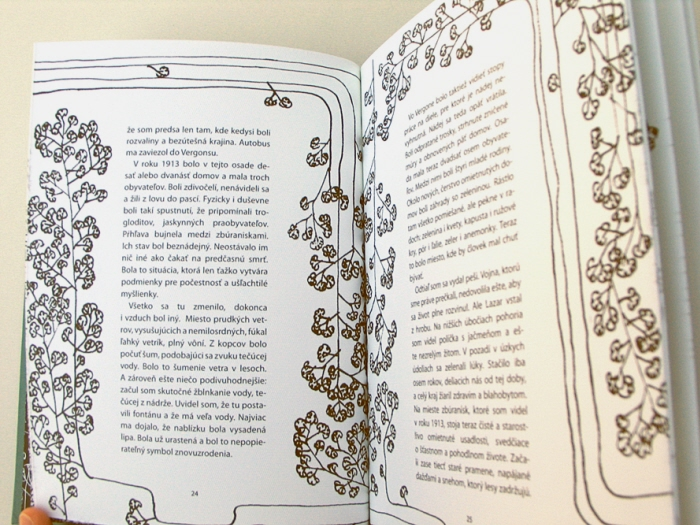spread - The idea of illustration in this book was follow the simple but strong tale: to grow,evolve and intesify with a story of book. As u can see on a first spreads, there are just seeds that at the end turned in to the forest, so the illustration does.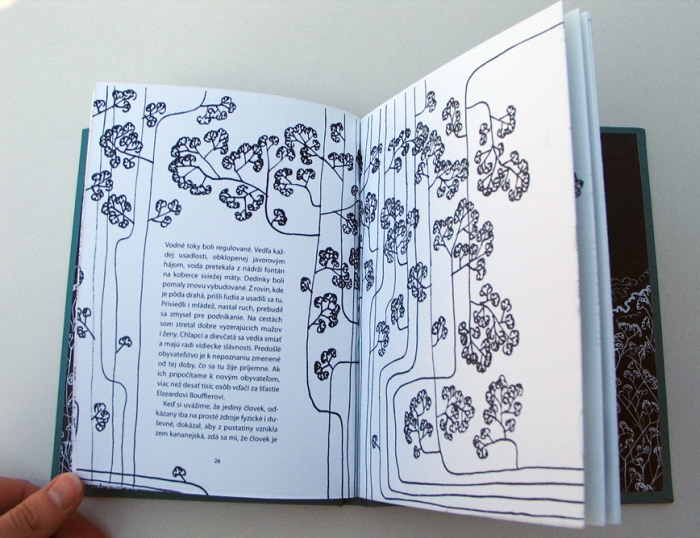spread - The idea of illustration in this book was follow the simple but strong tale: to grow,evolve and intesify with a story of book. As u can see on a first spreads, there are just seeds that at the end turned in to the forest, so the illustration does.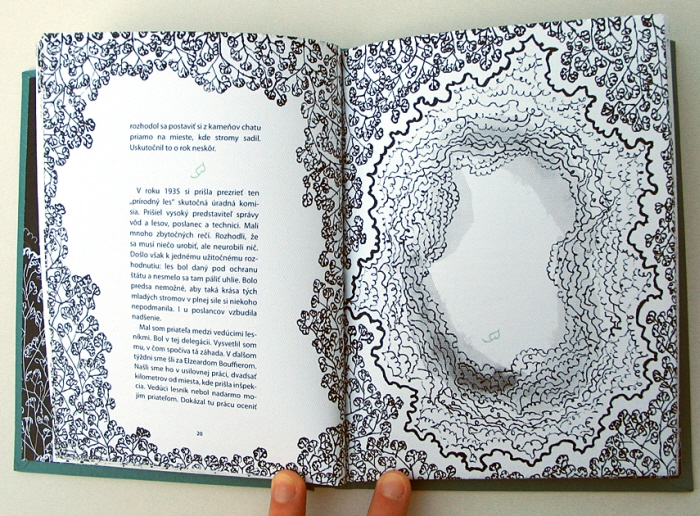spread - The idea of illustration in this book was follow the simple but strong tale: to grow,evolve and intesify with a story of book. As u can see on a first spreads, there are just seeds that at the end turned in to the forest, so the illustration does.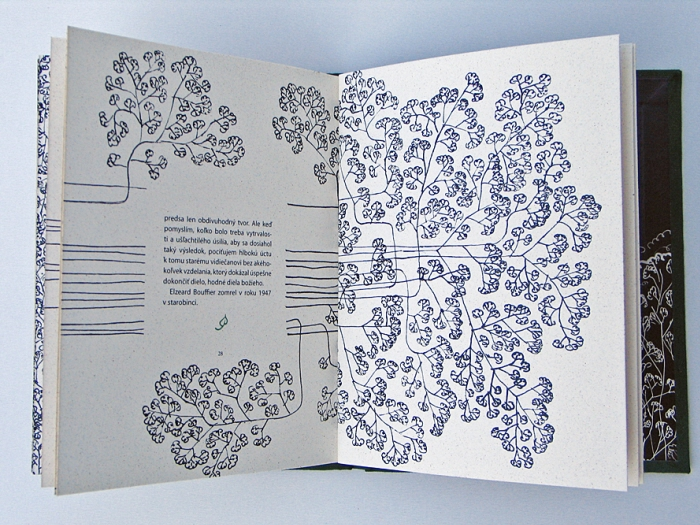spread - The idea of illustration in this book was follow the simple but strong tale: to grow,evolve and intesify with a story of book. As u can see on a first spreads, there are just seeds that at the end turned in to the forest, so the illustration does.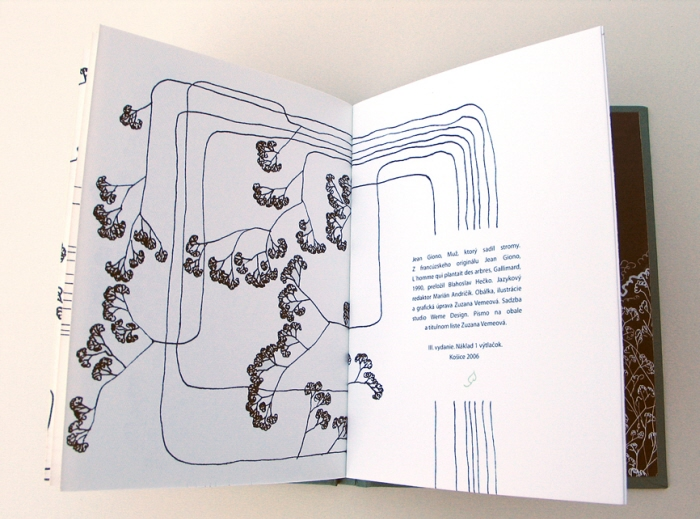imprint - The idea of illustration in this book was follow the simple but strong tale: to grow,evolve and intesify with a story of book. As u can see on a first spreads, there are just seeds that at the end turned in to the forest, so the illustration does.
• o
illustrated book The Man who planted the Trees